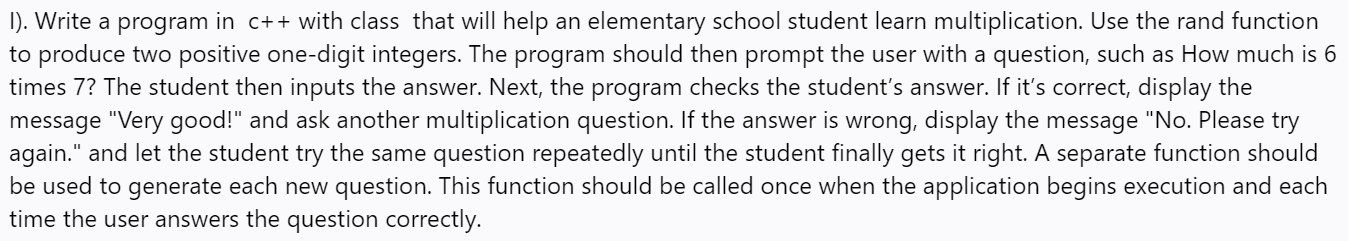# I). Write a program in c++ with class that will help an elementary school student learn multiplication. Use the rand functionto produce two positive one-digit integers. The program should then prompt the user with a question, such as How much is 6times 7? The student then inputs the answer. Next, the program checks the student's answer. If it's correct, display themessage "Very good!" and ask another multiplication question. If the answer is wrong, display the message "No. Please tryagain." and let the student try the same question repeatedly until the student finally gets it right. A separate function shouldbe used to generate each new question. This function should be called once when the application begins execution and eachtime the user answers the question correctly.

Question
73 views

I). Write a program in  c++ with class  that will help an elementary school student learn multiplication. Use the rand function to produce two positive one-digit integers. The program should then prompt the user with a question, such as How much is 6 times 7? The student then inputs the answer. Next, the program checks the student’s answer. If it’s correct, display the message "Very good!" and ask another multiplication question. If the answer is wrong, display the message "No. Please try again." and let the student try the same question repeatedly until the student finally gets it right. A separate function should be used to generate each new question. This function should be called once when the application begins execution and each time the user answers the question correctly.help_outlineImage TranscriptioncloseI). Write a program in c++ with class that will help an elementary school student learn multiplication. Use the rand function to produce two positive one-digit integers. The program should then prompt the user with a question, such as How much is 6 times 7? The student then inputs the answer. Next, the program checks the student's answer. If it's correct, display the message "Very good!" and ask another multiplication question. If the answer is wrong, display the message "No. Please try again." and let the student try the same question repeatedly until the student finally gets it right. A separate function should be used to generate each new question. This function should be called once when the application begins execution and each time the user answers the question correctly. fullscreen
check_circle

Step 1

A C++ program for the practice of multiplication is given as follows,

File name: “main.cpp”

#include <iostream>

#include <cmath>

#include <cstdlib>

//Decclare the method

//Declare namespace

using namespace std;

//Define the main() function

int main ()

{

//Declare the variables

int userNum,num;

//Call the method to get the multiplied value

//Execute while true

while(true)

{

//Get the answer input from the user

cin>>userNum;

//Check whether the user answer and the multipied value is same

if(userNum==num){

//Print the message

cout<<"Very good!"<<endl;

}

//Otherwise

else

{

//Print the message

}

}

}

//Define the method

//Generate two random numbers

int rand_int1 =(rand() %10);

int rand_int2 = (rand() %10);

cout<<"How much is "<< rand_int1<<  " times "<<rand_int2<<  "?"<<endl;

//Return the multiplied value

return rand_int1*rand_int2;

}

Screenshot of program #1:

Step 2

Screenshot of program #2:

Step 3

Screenshot of program #3...

### Want to see the full answer?

See Solution

#### Want to see this answer and more?

Solutions are written by subject experts who are available 24/7. Questions are typically answered within 1 hour.*

See Solution
*Response times may vary by subject and question.
Tagged in

### Computer Science